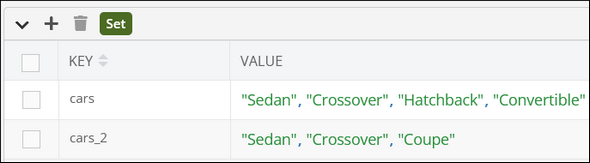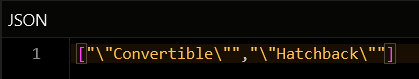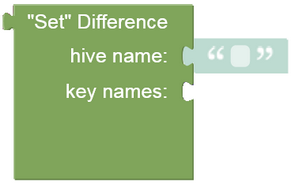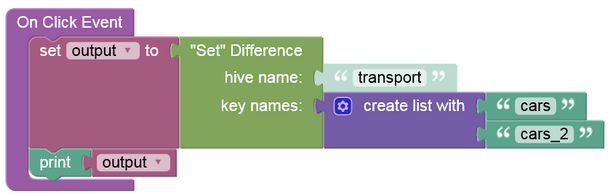# Difference¶

### Description¶

This operation finds the difference between the primary (first) set and all other specified sets. To find the difference, the operation subtracts identical values across multiple sets and returning the remainder (i.e. the difference) in a new set. The first set in the request is considered the main set whose remainder is left after the subtraction operation.

### Method¶

``````Backendless.Hive(hiveName).SetStore.difference(keyNames): Promise<values[]>;
``````

where:

Argument                Description
`hiveName` Name of a hive where the operation is performed. String value.
`keyNames` An array containing key names each identifying a set. Must be an array of string values.

### Return Value¶

An array containing values retrieved after the primary and all other consecutive sets are subtracted. Each individual value is of the any data type.

### Example¶

The example below subtracts all identical values and returns a new set containing the remainder.

``````await Backendless.Hive('transport').SetStore.difference(['cars', 'cars_2'])
``````

where:

Argument                Description
`'transport'` Name of a hive where the operation is performed.
`['cars', 'cars_2']` An array of key names each identifying a set containing multiple values.

Contents Of Specified SetsResponse### Codeless Reference¶where:

Argument                Description
`hive name` Name of a hive where the operation is performed.
`key names` A list containing key names identifying unique sets.

Returns a list containing values retrieved after the primary and all other consecutive sets are subtracted.

Consider the following Set storages:The example below subtracts items in the following sets: `"cars"` and `"cars_2"`; and gets the remainder:The output will look as shown below after the Codeless logic runs. Unique items(i.e. `"Coupe"`) that are outside of the primary set(`"cars"`) are skipped.